JEE  >  Area of Bounded Region

# Area of Bounded Region - Mathematics (Maths) Class 12 - JEE

The space occupied by the curve along with the axis, under the given condition is called area of bounded region.

(i) The area bounded by the curve y = F(x) above the X-axis and between the lines x = a, x = b is given by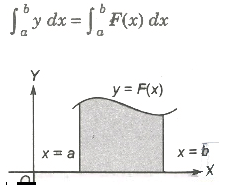(ii) If the curve between the lines x = a, x = b lies below the X-axis, then the required area is given by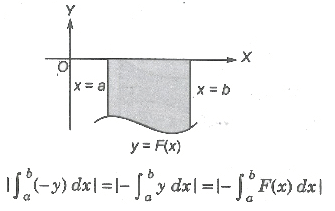(iii) The area bounded by the curve x = F(y) right to the Y-axis and the lines y = c, y = d is given by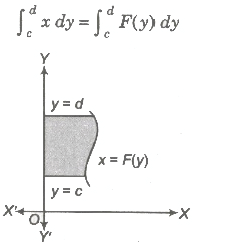(iv) If the curve between the lines y = c, y = d left to the Y-axis, then the area is given by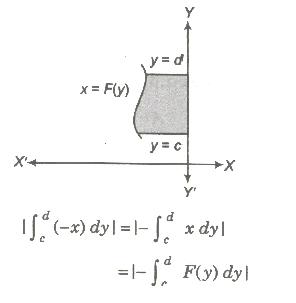(v) Area bounded by two curves y = F (x) and y = G (x) between x = a and x = b is given by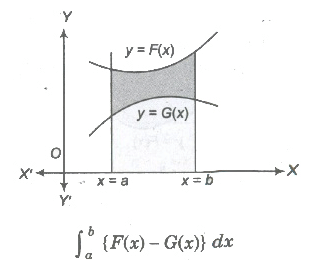(vi) Area bounded by two curves x = f(y) and x = g(y) between y=c and y=d is given by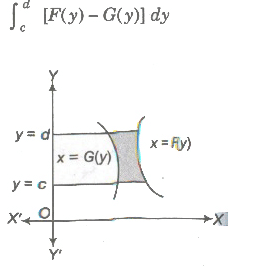(vii) If F (x) ≥. G (x) in [a, c] and F (x) ≤ G (x) in [c,d], where a < c < b, then area of the region bounded by the curves is given as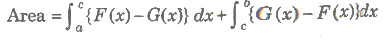Area of Curves Given by Polar Equations

Let  f(θ) be a continuous function,  θ ∈ (a, α), then the are t bounded by the curve r = f(θ) and radius α, β(α < β) is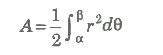Area of Parametric Curves

Let x = φ(t) and y = ψ(t) be two parametric curves, then area bounded by the curve, X-axis and ordinates x = φ(t1), x = ψ(t2) is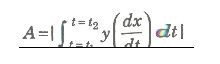Volume and Surface Area

If We revolve any plane curve along any line, then solid so generated is called solid of revolution.

1. Volume of Solid Revolution

1. The volume of the solid generated by revolution of the area bounded by the curve y = f(x), the axis of x and the ordinates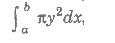it being given that f(x) is a continuous a function in the interval (a, b).

2. The volume of the solid generated by revolution of the area bounded by the curve x = g(y), the axis of y and two abscissas y = c and y = d is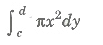it being given that g(y) is a continuous function in the interval (c, d).

Surface of Solid Revolution

(i) The surface of the solid generated by revolution of the area bounded by the curve y = f(x), the axis of x and the ordinates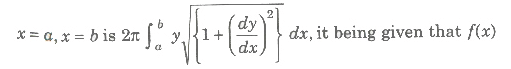is a continuous function in the interval (a, b).

(ii) The surface of the solid generated by revolution of the area bounded by the curve x = f (y), the axis of y and y = c, y = d is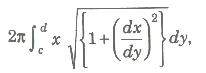continuous function in the interval (c, d).

Curve Sketching

1. symmetry

1. If powers of y in a equation of curve are all even, then curve is symmetrical about X-axis.
2. If powers of x in a equation of curve are all even, then curve is symmetrical about Y-axis.
3. When x is replaced by -x and y is replaced by -y, then curve is symmetrical in opposite quadrant.
4. If x and y are interchanged and equation of curve remains unchanged curve is symmetrical about line y = x.

2. Nature of Origin

1. If point (0, 0) satisfies the equation, then curve passes through origin.
2. If curve passes through origin, then equate low st degree term to zero and get equation of tangent. If there are two tangents, then origin is a double point.

3. Point of Intersection with Axes

1. Put y = 0 and get intersection with X-axis, put x = 0 and get intersection with Y-axis.
2. Now, find equation of tangent at this point i. e. , shift origin to the point of intersection and equate the lowest degree term to zero.
3. Find regions where curve does not exists. i. e., curve will not exit for those values of variable when makes the other imaginary or not defined.

4. Asymptotes

1. Equate coefficient of highest power of x and get asymptote parallel to X-axis.
2. Similarly equate coefficient of highest power of y and get asymptote parallel to Y-axis.

5. The Sign of (dy/dx)

Find points at which (dy/dx) vanishes or becomes infinite. It gives us the points where tangent is parallel or perpendicular to the X-axis.

6. Points of Inflexion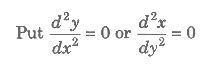and solve the resulting equation.If some point of inflexion is there, then locate it exactly.

Taking in consideration of all above information, we draw an approximate shape of the curve.

Shape of Some Curves is Given Below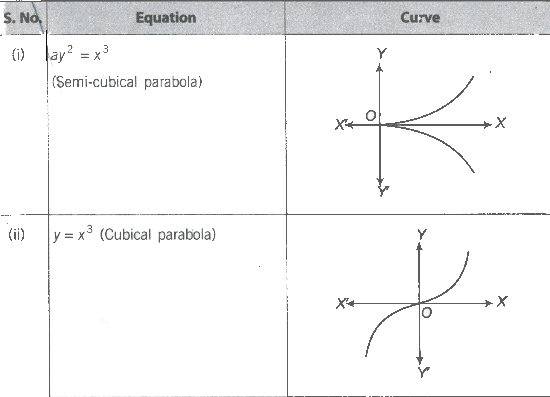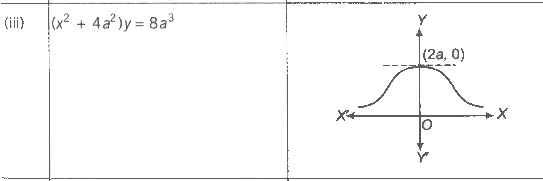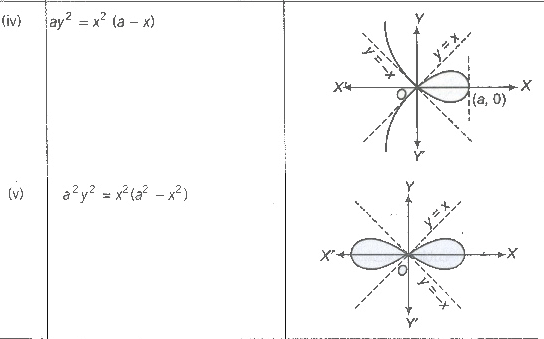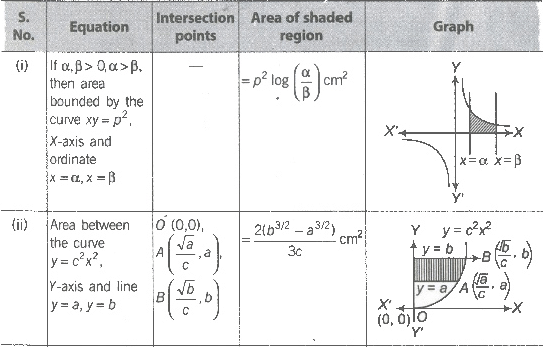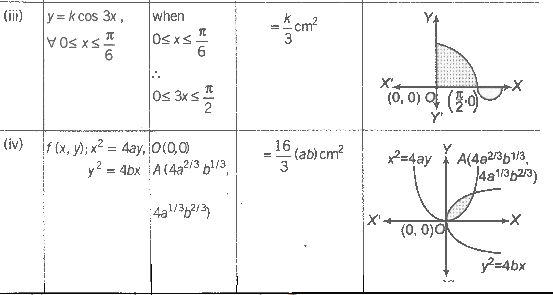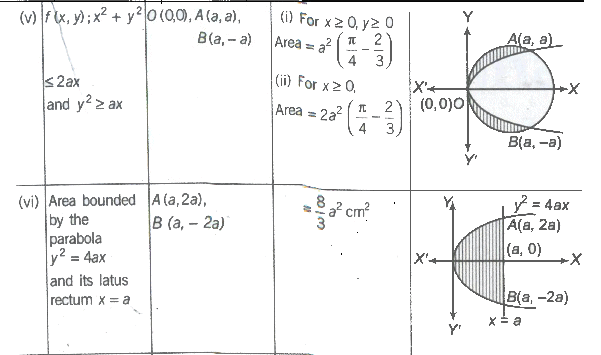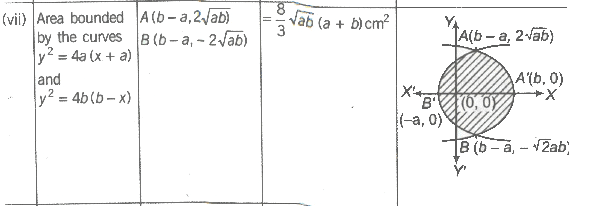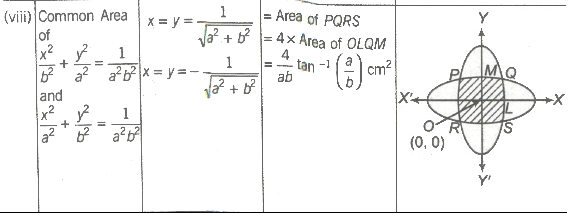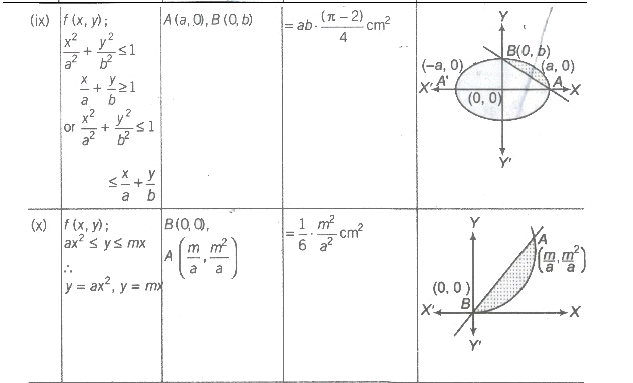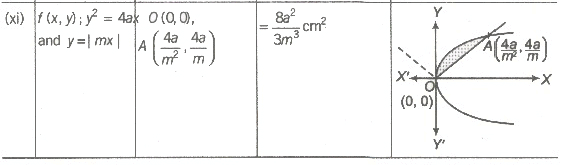The document Area of Bounded Region | Mathematics (Maths) Class 12 - JEE is a part of the JEE Course Mathematics (Maths) Class 12.
All you need of JEE at this link: JEE

## FAQs on Area of Bounded Region - Mathematics (Maths) Class 12 - JEE

 1. What is the formula to calculate the area of a bounded region?Ans. The formula to calculate the area of a bounded region depends on the shape of the region. For simple shapes like rectangles or squares, the area can be calculated by multiplying the length and width. For more complex shapes, the area can be determined using formulas specific to each shape, such as the formula for the area of a circle (πr²) or the formula for the area of a triangle (1/2 base × height).
 2. How can I find the area of a bounded region with irregular shapes?Ans. To find the area of a bounded region with irregular shapes, you can use the method of dividing the shape into smaller, more manageable shapes. Break down the irregular shape into simpler shapes like rectangles, triangles, or circles. Then, calculate the area of each individual shape using the appropriate formula and sum up all the areas to find the total area of the bounded region.
 3. Can the area of a bounded region be negative?Ans. No, the area of a bounded region cannot be negative. Area is always a positive value, representing the measure of the surface enclosed by the boundary of a shape or region. It is a measure of how much space the shape or region occupies, and therefore, it cannot have a negative value.
 4. What is the importance of calculating the area of a bounded region?Ans. Calculating the area of a bounded region is important for various reasons. It is used in many practical applications, such as architecture, construction, landscaping, and engineering. Knowing the area of a bounded region helps in determining the amount of materials needed, estimating costs, planning layouts, and designing structures. It also allows for accurate measurement and comparison of different regions or shapes.
 5. Can the area of a bounded region be infinite?Ans. No, the area of a bounded region cannot be infinite. A bounded region, by definition, has a finite boundary that encloses a finite area. Infinite areas are associated with unbounded regions, such as infinitely extending lines or planes. However, when dealing with practical applications or real-world scenarios, bounded regions always have a finite area.

## Mathematics (Maths) Class 12

208 videos|243 docs|139 tests

## Mathematics (Maths) Class 12

208 videos|243 docs|139 tests
Signup to see your scores go up within 7 days! Learn & Practice with 1000+ FREE Notes, Videos & Tests.
10M+ students study on EduRev
Track your progress, build streaks, highlight & save important lessons and more!(Scan QR code)
Related Searches

,

,

,

,

,

,

,

,

,

,

,

,

,

,

,

,

,

,

,

,

,

;